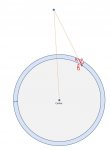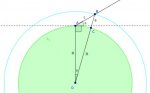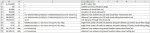# Satellite line of site, cloud obstruction

#### brandonnotgoodatmath

##### New member
Hi all,

I am new to this forum and looking for some help. I have a task to determine the amount of cloud cover a signal will encounter from a satellite ground station to asatellite. I need to determine the formula so that I can check for multiple locations. Any help would be much appreciated!

I imagine a point on earth (say in Munich), pointing towards a satellite with an azimuth of 120 (degrees) and an elevation of 30 (degrees). Cloud cover for this area is from ground level to 10000m (making this up as I go). So the earth is one circle, with the could cover being a slightly larger circle, and I would like to find all sides of the triangle. The hyp. from the originating point through the cloud cover to the point when it exits the clouds would be leg "C". The distance from the top of the clouds to the earths surface is know at 10000m and will be leg "A". And the originating point to point on earth directly below where the signal exits the could cover will be leg "B". See the illustration.

I'm also looking for the coordinates of the point AB.

Summary:

Circle 1 = Earths radius
Circle 2 = Could cover radius (10000m larger than earth)
Originating point = BC (Lat/Lon:47°59'44.8"N 11°07'17.3"E) <-- Yes, I picked a point on a lakeExit clouds point = AC
Point below exit = AB
Angle of BC = 30 (deg)
Angle of AB = 90 (deg)
Azimuth = 120 (deg) don't think this is relevant other than to find lat/lon of point AB

Thank you in advance!!!

Brandon

#### Dr.Peterson

##### Elite Member
Hi all,

I am new to this forum and looking for some help. I have a task to determine the amount of cloud cover a signal will encounter from a satellite ground station to aView attachment 10820 satellite. I need to determine the formula so that I can check for multiple locations. Any help would be much appreciated!

I imagine a point on earth (say in Munich), pointing towards a satellite with an azimuth of 120 (degrees) and an elevation of 30 (degrees). Cloud cover for this area is from ground level to 10000m (making this up as I go). So the earth is one circle, with the could cover being a slightly larger circle, and I would like to find all sides of the triangle. The hyp. from the originating point through the cloud cover to the point when it exits the clouds would be leg "C". The distance from the top of the clouds to the earths surface is know at 10000m and will be leg "A". And the originating point to point on earth directly below where the signal exits the could cover will be leg "B". See the illustration.

I'm also looking for the coordinates of the point AB.

Summary:

Circle 1 = Earths radius
Circle 2 = Could cover radius (10000m larger than earth)
Originating point = BC (Lat/Lon:47°59'44.8"N 11°07'17.3"E) <-- Yes, I picked a point on a lakeExit clouds point = AC
Point below exit = AB
Angle of BC = 30 (deg)
Angle of AB = 90 (deg)
Azimuth = 120 (deg) don't think this is relevant other than to find lat/lon of point AB

Thank you in advance!!!

Brandon
First, your notation is confusing. (Maybe it is standard in your area, but I'm not aware of that.) You are using capital letters A, B, C to stand for the sides of the triangle, where we typically use a, b, c; but we never use a pair of letters like BC to stand for a point! I suppose you mean the point where sides B and C meet. Traditionally, we'd call the points A, B, and C, so that for example the side you call A is what we call side a, and point A is the opposite vertex, where sides b and c meet. So for my sanity, I'm going to rewrite your summary:

Circle 1 = Earth's radius
Circle 2 = Cloud cover radius (10000m larger than earth)
Originating point = A
Exit clouds point = B
Point below exit = C
Angle at A = 30 (deg)
Angle at C = 90 (deg)
Height BC = a = 10 000 m

The sphericity of the earth will have little effect unless you have a very shallow angle, so you could use ordinary right triangle trigonometry. In this case, sin(A) = a/c, so that 10 000/c = sin(A), and c = 10 000/sin(A). There's your formula for the distance through the clouds. (Leave a in place of 10 000 for the general formula.)

For the horizontal distance AC = b, we have tan(A) = a/b, so b = a/tan(A). To find the latitude and longitude of point C, you'll have to look up appropriate formulas based on the location of A, direction, and distance b. That's a separate question, and is answered in many places.

Now, if you are going to have shallower angles than in the example, so that distance b gets relatively large, then you would have to take curvature into effect; we can deal with that if you find it necessary. I suppose you'll also want to deal with the case where clouds don't reach the ground, unless it is always foggy there.

#### brandonnotgoodatmath

##### New member
Thank you Dr. Peterson!

Clearly, you can see I need a lot of help.

I do need to take the curvature of the earth into account. I will be looking at locations with very low angles, and cloud cover that goes much higher.

Regarding the low clouds, I'm not sure. I will be working with radar data. I haven't had a chance to review the data yet, so I do not yet know if I'll have elevations where the clouds (reflectivity) start and stop. I think I will just have to rely on historic data for a particular area. But if the radar data provides that information, I guess I would have a third circle in the diagram and need to measure the length of the signal between the cloud lower limit and cloud upper limit.

Brandon

#### Dr.Peterson

##### Elite Member
Thank you Dr. Peterson!

Clearly, you can see I need a lot of help.

I do need to take the curvature of the earth into account. I will be looking at locations with very low angles, and cloud cover that goes much higher.

Regarding the low clouds, I'm not sure. I will be working with radar data. I haven't had a chance to review the data yet, so I do not yet know if I'll have elevations where the clouds (reflectivity) start and stop. I think I will just have to rely on historic data for a particular area. But if the radar data provides that information, I guess I would have a third circle in the diagram and need to measure the length of the signal between the cloud lower limit and cloud upper limit.

Brandon
Our usual purpose is to help students learn to solve problems themselves; your "notgoodatmath" (which is a very common claim) almost leads me to want to force you to do the work yourself, so you can see that you can do it. But this one is too hard to try to lead you through by hints, so I'll just give you the conclusion of my work (and others who are reading along can ask me about it if they want). The fact that I'm leaving some things to do that you are not complaining about tells me that I'm right that you can do more than you're admitting ...

Here's a picture of the round-earth version:I used the law of cosines to solve for c:

$$\displaystyle c = -R \sin\alpha + \sqrt{R^2 \sin^2\alpha + 2Ra + a^2}$$

This worked correctly for the measurements in my drawing (made with Geogebra). We could also have used the law of sines, but that's more indirect.

The distance AC is best described in terms of angle theta; using the law of sines, we can get

$$\displaystyle \sin\theta = \dfrac{c\cos\alpha}{R + a}$$

The distance is then R times theta (in radians).

If you had the bottom of the clouds, you could just repeat the process and subtract.

#### brandonnotgoodatmath

##### New member
Thank you very much Dr. Peterson. It took me a few iterations in Excel to get the formula entered correctly, and about 6 hours of goofing around on GeoGebra (awesome by the way) to see the formula work. My drawing did not come out as clean as yours did, maybe it will get better with practice.

In case anyone else is looking for the Excel formulas:

Code:
[TABLE="width: 1078"]
[TR]
[TD="align: right"]6,371,000[/TD]
[TD]  R[/TD]
[TD]---[/TD]
[TD="colspan: 7"]earth's radius (m)[/TD]
[/TR]
[TR]
[TD="align: right"]30[/TD]
[TD]  α[/TD]
[TD]---[/TD]
[TD="colspan: 7"]satellite antenna elevation (deg)[/TD]
[/TR]
[TR]
[TD="align: right"]20,000[/TD]
[TD]  a[/TD]
[TD]---[/TD]
[TD="colspan: 7"]elevation of cloud floor (m)[/TD]
[/TR]
[TR]
[TD="align: right"]50,000[/TD]
[TD]  b[/TD]
[TD]---[/TD]
[TD="colspan: 7"]elevation of cloud ceiling (m)[/TD]
[/TR]
[TR]
[TD="align: right"]39,813.98[/TD]
[TD]  c[/TD]
[TD][SIZE=1]=-A1*SIN(RADIANS(A2))+SQRT((A1^2*SIN(RADIANS(A2))^2)+(2*A1*A3)+(A3^2))[/SIZE][/TD]
[TD="colspan: 7"]distance from antenna to LOS and cloud floor intersection (m)[/TD]
[/TR]
[TR]
[TD="align: right"]98,858.42[/TD]
[TD]  d[/TD]
[TD][SIZE=1]=-A1*SIN(RADIANS(A2))+SQRT((A1^2*SIN(RADIANS(A2))^2)+(2*A1*A4)+(A4^2))[/SIZE][/TD]
[TD="colspan: 7"]distance from antenna to LOS and cloud ceiling intersection (m)[/TD]
[/TR]
[TR]
[TD="align: right"]59,044.45[/TD]
[TD]  e[/TD]
[TD]=A6-A5[/TD]
[TD="colspan: 7"]LOS distance through the cloud (m)[/TD]
[/TR]
[TR]
[TD="align: right"]0.31[/TD]
[TD]  θ[SUB]1[/SUB][/TD]
[TD][SIZE=1]=DEGREES(ACOS((A1^2 + (A1+A3)^2-A5^2)/(2*A1*(A1+A3))))[/SIZE][/TD]
[TD="colspan: 7"]angle from center of earth to antenna and point where LOS enters cloud floor (deg)[/TD]
[/TR]
[TR]
[TD="align: right"]0.76[/TD]
[TD]  θ[SUB]2[/SUB][/TD]
[TD][SIZE=1]=DEGREES(ACOS((A1^2 + (A1+A4)^2-A6^2)/(2*A1*(A1+A4))))[/SIZE][/TD]
[TD="colspan: 7"]angle from center of earth to antenna and point where LOS exits cloud ceiling (deg)[/TD]
[/TR]
[TR]
[TD="align: right"]34,372.18[/TD]
[TD]  dist[SUB]1[/SUB][/TD]
[TD]=A1*RADIANS(A8)[/TD]
[TD="colspan: 7"]distance from antenna to ground location below cloud floor (m)[/TD]
[/TR]
[TR]
[TD="align: right"]84,949.75[/TD]
[TD]  dist[SUB]2[/SUB][/TD]
[TD]=A1*RADIANS(A9)[/TD]
[TD="colspan: 7"]distance from antenna to ground location below cloud ceiling (m)[/TD]
[/TR]
[/TABLE]If anyone finds a mistake in my formulas or labeling please let me know.

#### Dr.Peterson

##### Elite Member
Thank you very much Dr. Peterson. It took me a few iterations in Excel to get the formula entered correctly, and about 6 hours of goofing around on GeoGebra (awesome by the way) to see the formula work. My drawing did not come out as clean as yours did, maybe it will get better with practice.

In case anyone else is looking for the Excel formulas:

Code:
[TABLE="width: 1078"]
[TR]
[TD="align: right"]6,371,000[/TD]
[TD]  R[/TD]
[TD]---[/TD]
[TD="colspan: 7"]earth's radius (m)[/TD]
[/TR]
[TR]
[TD="align: right"]30[/TD]
[TD]  α[/TD]
[TD]---[/TD]
[TD="colspan: 7"]satellite antenna elevation (deg)[/TD]
[/TR]
[TR]
[TD="align: right"]20,000[/TD]
[TD]  a[/TD]
[TD]---[/TD]
[TD="colspan: 7"]elevation of cloud floor (m)[/TD]
[/TR]
[TR]
[TD="align: right"]50,000[/TD]
[TD]  b[/TD]
[TD]---[/TD]
[TD="colspan: 7"]elevation of cloud ceiling (m)[/TD]
[/TR]
[TR]
[TD="align: right"]39,813.98[/TD]
[TD]  c[/TD]
[TD][SIZE=1]=-A1*SIN(RADIANS(A2))+SQRT((A1^2*SIN(RADIANS(A2))^2)+(2*A1*A3)+(A3^2))[/SIZE][/TD]
[TD="colspan: 7"]distance from antenna to LOS and cloud floor intersection (m)[/TD]
[/TR]
[TR]
[TD="align: right"]98,858.42[/TD]
[TD]  d[/TD]
[TD][SIZE=1]=-A1*SIN(RADIANS(A2))+SQRT((A1^2*SIN(RADIANS(A2))^2)+(2*A1*A4)+(A4^2))[/SIZE][/TD]
[TD="colspan: 7"]distance from antenna to LOS and cloud ceiling intersection (m)[/TD]
[/TR]
[TR]
[TD="align: right"]59,044.45[/TD]
[TD]  e[/TD]
[TD]=A6-A5[/TD]
[TD="colspan: 7"]LOS distance through the cloud (m)[/TD]
[/TR]
[TR]
[TD="align: right"]0.31[/TD]
[TD]  θ[SUB]1[/SUB][/TD]
[TD][SIZE=1]=DEGREES(ACOS((A1^2 + (A1+A3)^2-A5^2)/(2*A1*(A1+A3))))[/SIZE][/TD]
[TD="colspan: 7"]angle from center of earth to antenna and point where LOS enters cloud floor (deg)[/TD]
[/TR]
[TR]
[TD="align: right"]0.76[/TD]
[TD]  θ[SUB]2[/SUB][/TD]
[TD][SIZE=1]=DEGREES(ACOS((A1^2 + (A1+A4)^2-A6^2)/(2*A1*(A1+A4))))[/SIZE][/TD]
[TD="colspan: 7"]angle from center of earth to antenna and point where LOS exits cloud ceiling (deg)[/TD]
[/TR]
[TR]
[TD="align: right"]34,372.18[/TD]
[TD]  dist[SUB]1[/SUB][/TD]
[TD]=A1*RADIANS(A8)[/TD]
[TD="colspan: 7"]distance from antenna to ground location below cloud floor (m)[/TD]
[/TR]
[TR]
[TD="align: right"]84,949.75[/TD]
[TD]  dist[SUB]2[/SUB][/TD]
[TD]=A1*RADIANS(A9)[/TD]
[TD="colspan: 7"]distance from antenna to ground location below cloud ceiling (m)[/TD]
[/TR]
[/TABLE]
View attachment 10824

If anyone finds a mistake in my formulas or labeling please let me know.
Looks good to me (without digging too deeply). I was right that you didn't need more than I gave you.